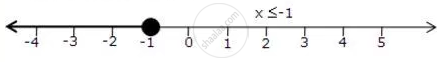Share

# Represent the Solution of the Given Inequalities on the Real Number Line 7 - X <= 2 - 6x - Mathematics

Course

#### Question

Represent the solution of the given inequalities on the real number line

7 - x <= 2 - 6x

#### Solution

7 - x <= 2 - 6x

5x <= -5

x <= -1

The solution on number line is:Is there an error in this question or solution?